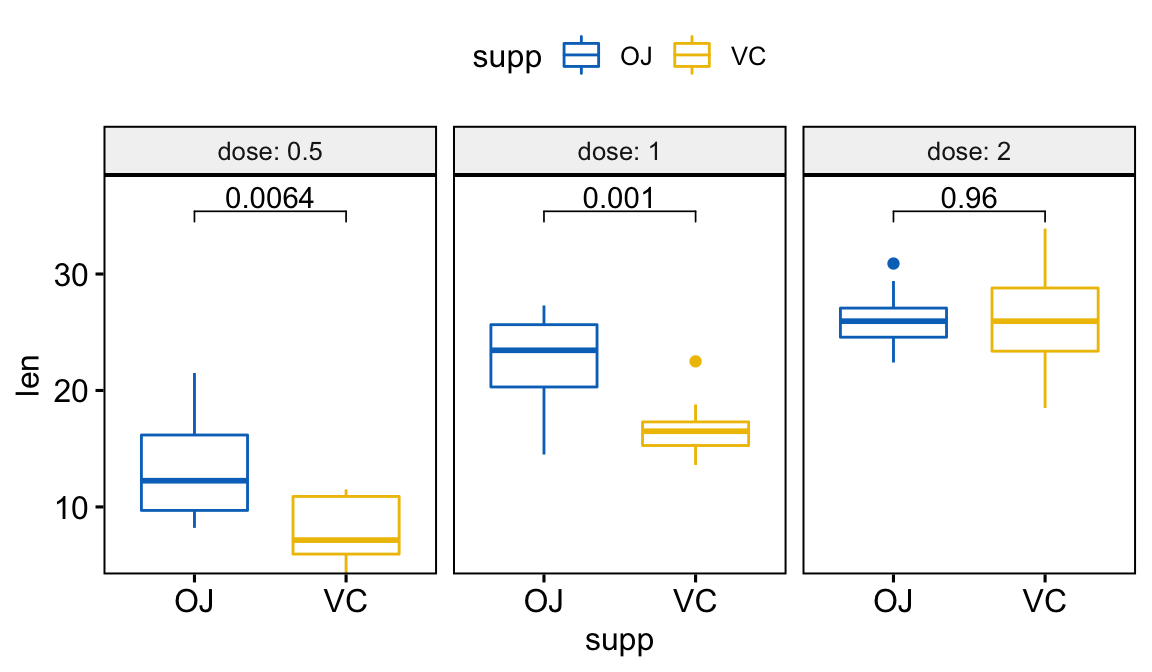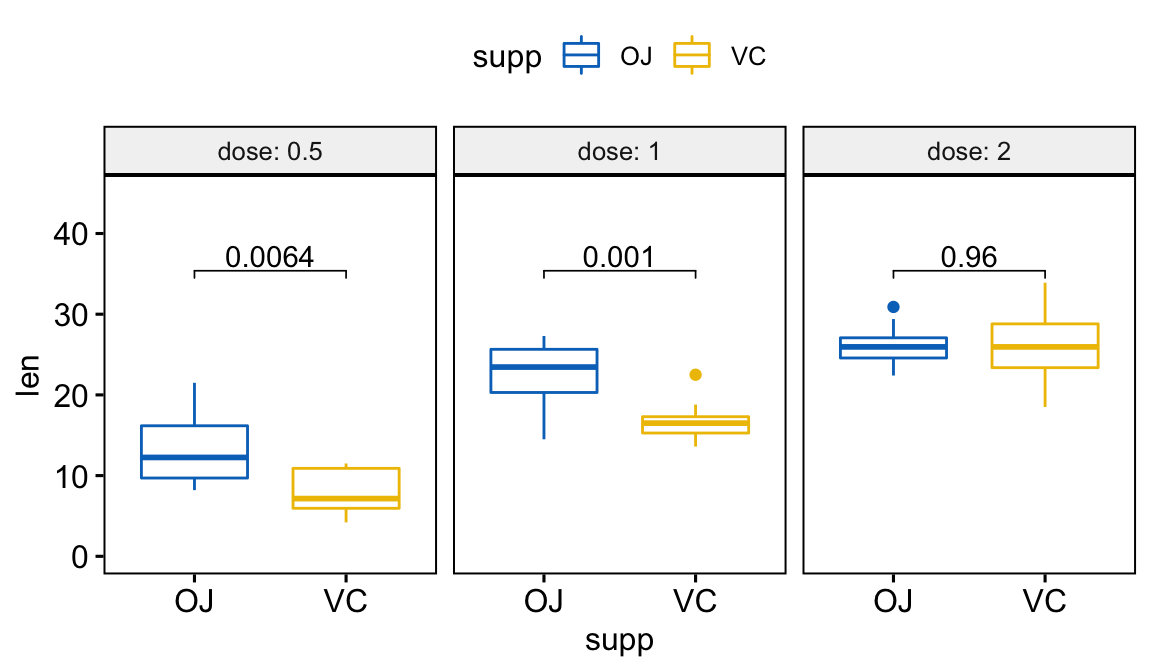# GGPLOT Facet: How to Add Space Between Labels on the Top of the Chart and the Plot Border#### GGPLOT Facet: How to Add Space Between Labels on the Top of the Chart and the Plot Border

This article describes how add space between the labels, on the top of the chart (bar plot, box plot, etc), and the plot border when using the ggplot2 facet functions (facet_wrap() and facet_grid()).

In the demo example, we’ll create a publication ready plot with p-values using the ggpubr package, an extension of ggplot2.

Concerning adding spaces between the labels and plot top margin, you will learn multiple solutions, such as:

1. Changing the y-axis limits
2. Expanding the y-axis scales using the combination of the ggplot2 functions scale_y_continuous() and expansion()

Contents:

## Create a faceted box plot with p-values labels

library(ggpubr)
p <- ggboxplot(
ToothGrowth, x = "supp", y = "len",
color = "supp", palette = "jco",
facet.by = "dose", short.panel.labs = FALSE
) +
stat_compare_means(
method = "t.test", label = "p.format",
comparisons=list(c("OJ","VC"))
)
pIt can be seen that, p-values are not shown completely. In the next section we will show how to add more spaces between the p-value labels and the plot top border.

## Solution 1: Expanding the y-axis using the ggplot2 function expansion()

Key R function:

expansion(mult = 0, add = 0)
• mult: vector of multiplicative range expansion factors. If length 1, both the lower and upper limits of the scale are expanded outwards by the mult factor. If length 2, the lower limit is expanded by mult and the upper limit by mult factors.
• add: vector of additive range expansion constants. If length 1, both the lower and upper limits of the scale are expanded outwards by add units. If length 2, the lower limit is expanded by add and the upper limit by add constants.
# No space below the box plots
# Add 10% space on the y-axis above the box plots
p +
scale_y_continuous(expand = expansion(mult = c(0, 0.1)))## Solution 2: Using the ggplot2 function ylim()

This is only useful if the scales are similar for the different facet panels.

p + ylim (c(0, 45))## Conclusion

This article describes how to add space between labels and the ggplot top border when using the facet functions. This can be easily achieved using the combinations of scale_y_continuous() and expansion(). Note that it’s also possible to combine scale_x_continuous() and expansion() for adding spaces between data and the x-axis.

### Comment ( 1 )

•Kamau Lindhardt

OMG 😱 Thank you so much!! This saved my day. Amazing 👏